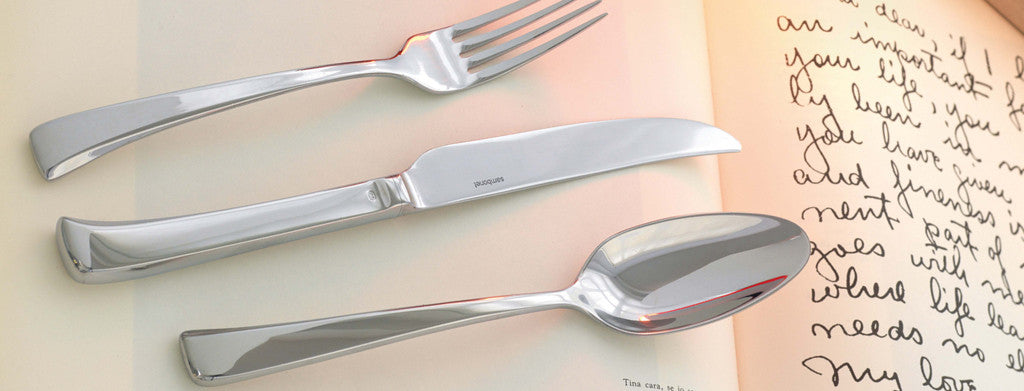Shopping Cart

### Refine

View all

#### Price# Cutlery£5.48 each (ex VAT)£37.23 each (ex VAT)£6.15 each (ex VAT)£5.48 each (ex VAT)£3.46 each (ex VAT)£3.95 each (ex VAT)£7.57 each (ex VAT)£5.48 each (ex VAT)£3.95 each (ex VAT)£8.70 each (ex VAT)£3.95 each (ex VAT)£8.87 each (ex VAT)£6.15 each (ex VAT)£7.57 each (ex VAT)£2.99 each (ex VAT)£4.98 each (ex VAT)£9.17£3.95 each (ex VAT)£6.15 each (ex VAT)£6.76 each (ex VAT)£12.24£5.48 each (ex VAT)£3.46 each (ex VAT)£36.80 each (ex VAT)£8.70 each (ex VAT)£5.07 each (ex VAT)£6.61 each (ex VAT)£5.80 each (ex VAT)£8.70 each (ex VAT)£7.74 each (ex VAT)£7.74 each (ex VAT)£7.74 each (ex VAT)£6.44 each (ex VAT)£5.68 each (ex VAT)£15.16£9.51 each (ex VAT)£12.24£7.39 each (ex VAT)£29.00£13.54 each (ex VAT)£7.74 each (ex VAT)£25.77 each (ex VAT)£7.74 each (ex VAT)£6.15 each (ex VAT)£12.10£8.70 each (ex VAT)£12.51 each (ex VAT)£10.80 each (ex VAT)